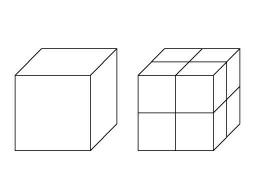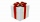# Cube basics

How long is the edge length of a cube with volume 15 m3?

x =  2.47 m

### Step-by-step explanation:Did you find an error or inaccuracy? Feel free to write us. Thank you!Tips to related online calculators
Tip: Our volume units converter will help you with the conversion of volume units.

## Related math problems and questions:

• Cube V2SThe volume of the cube is 27 dm cubic. Calculate the surface of the cube.
• The cubeThe cube has a surface area of 486 m ^ 2. Calculate its volume.
• Cube 3How many times will increase the volume of a cube if we double the length of its edge?
• The cubeThe cube has a surface of 600 cm2. What is its volume?
• Length of the edgeFind the length of the edge of a cube with a cm2 surface and a volume in cm3 expressed by the same number.
• Volume per timeHow long does fill take for a pump with a volume flow of 200 l per minute fill a cube-shaped tank up to 75% of its height if the length of the cube edge is 4 m?
• Edges or sidesCalculate the cube volume, if the sum of the lengths of all sides is 276 cm.
• Cube surface and volumeFind the surface of the cube with a volume of 27 dm3.
• Cube edgeDetermine the edges of the cube when the surface is equal to 37.5 cm square.How much wrapping paper is needed to wrap a cube-shaped gift with edge measuring 15 cm?
• Roof repairTo repair the roof, we need 15 pieces of boards 6 m long, 15 cm wide and 25 mm thick. How many euros will we pay for all boards if 1 m3 of boards costs 500 euros?
• An exampleAn example is playfully for grade 6 from Math and I don't know how to explain it to my daughter when I don't want to use the calculator to calculate the cube root. Thus: A cuboid was made from a block of 16x18x48 mm of modeline. What will be the edge of t
• Third dimensionCalculate the third dimension of the cuboid: a) V = 224 m3, a = 7 m, b = 4 m b) V = 216 dm3, a = 9 dm, c = 4 dm
• Cube rootFor 13, Sam wrote 2891 instead of the correct cube number. By how much was he wrong?
• A plasticineJožko modeled from plasticine. He used 27g of plasticine to model a 3 cm long cube. How many grams of plasticine will it need to mold cubes with an edge of 6cm?
• Cube into cylinderIf we dip a wooden cube into a barrel with a 40cm radius, the water will rise 10 cm. What is the size of the cube edge?
• Tetrahedral prismThe height of a regular tetrahedral prism is three times greater than the length of the base edge. Calculate the length of the base edge, if you know that the prism volume is 2187 cm3.